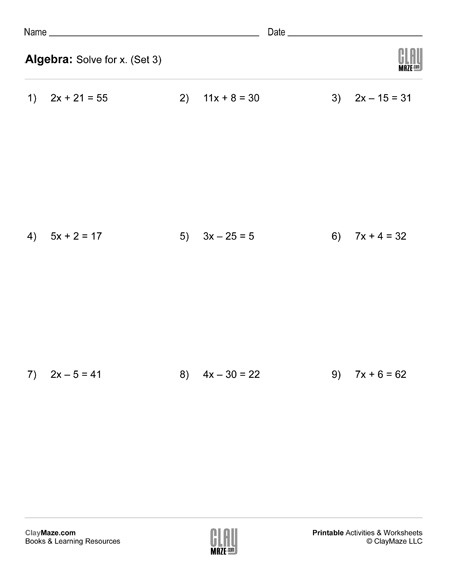# Solve the Equations I (Set 3)

Print this practice worksheet for students learning to solve equations. Each problem has a combination of multiplication and either addition or subtraction. Division will also be used to find the final answer.